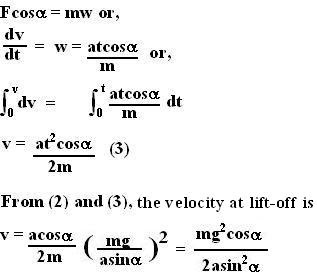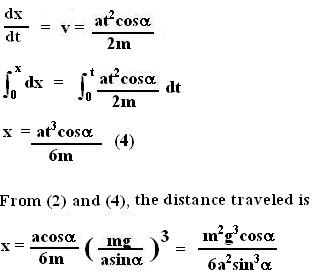## Tuesday, August 7, 2007

### Irodov Problem 1.68The force diagram is shown beside. There are three forces acting in the vertical direction, i) the force of gravity mg, ii) the normal reaction from the surface N and iii) the component of the force F acting along the vertical direction. Before the body lifts off the floor, there is no acceleration of the body in the vertical direction, thus,At the instant when the body looses contact with the ground, the normal reaction N must become 0 (since there is no contact with the ground). Thus, setting N=0 in (1) we for lift-off condition we have,Now let us consider the motion of the body along the horizontal direction. There is only one force acting on the body along this direction - the component of F namely. If the instantenous acceleration of the body is w, then,Again from (3), if x is the distance traveled by the mass at any given instant, we can write,Anonymous said...

Why can't I say:
F cosx = m*acc
=>acc = FcosX/m
=>acc = at*cosX/m

Then v = u + at. u = 0
Hence v = (at * cosX/m) * t

Substitute for t from equation 2.
It gives a different answer. Can you explain why ?

Krishna Kant Chintalapudi said...

The equation v = u + acc*t is correct only when the acceleration acc is constant with time not when acc is changing with time.

For example if acc = bt then,
v = bt^/2 + ut.

In this problem acc is changing with time.Anonymous said...

That was the key point I was missing. Should have figured it out since the problem says the force applied is a function of time and hence acceleration (F=ma) is a function of time too.
I suggest you include a one line explanation per the above in you solution for some of us who have this doubt.
Thanks Krishna. You have done a great job on this blog.Anonymous said...

When calculating distance traveled along the horizontal direction shouldn't we also include friction which would also be time dependent?

Force F also has a component along the vertical direction, so friction would be:

(mg - at sin (alpha))k

Krishna Kant Chintalapudi said...

Yes indeed there were friction what you are saying is correct - but the problem says "smooth surface" implying that there is no frictionAnonymous said...

i must say you have done most brilliant job on net. I could have never guessed that this is a case of variable acceleration on my own. Thanks.